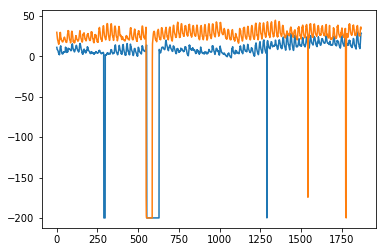# Time Series - Exponential Smoothing

In this chapter, we will talk about the techniques involved in exponential smoothing of time series.

## Simple Exponential Smoothing

Exponential Smoothing is a technique for smoothing univariate time-series by assigning exponentially decreasing weights to data over a time period.

Mathematically, the value of variable at time ‘t+1’ given value at time t, y_(t+1|t) is defined as −

$$y_{t+1|t}\:=\:\alpha y_{t}\:+\:\alpha\lgroup1 -\alpha\rgroup y_{t-1}\:+\alpha\lgroup1-\alpha\rgroup^{2}\:y_{t-2}\:+\:...+y_{1}$$

where,$0\leq\alpha \leq1$ is the smoothing parameter, and

$y_{1},....,y_{t}$ are previous values of network traffic at times 1, 2, 3, … ,t.

This is a simple method to model a time series with no clear trend or seasonality. But exponential smoothing can also be used for time series with trend and seasonality.

## Triple Exponential Smoothing

Triple Exponential Smoothing (TES) or Holt's Winter method, applies exponential smoothing three times - level smoothing $l_{t}$, trend smoothing $b_{t}$, and seasonal smoothing $S_{t}$, with $\alpha$, $\beta^{*}$ and $\gamma$ as smoothing parameters with ‘m’ as the frequency of the seasonality, i.e. the number of seasons in a year.

According to the nature of the seasonal component, TES has two categories −

• Holt-Winter's Additive Method − When the seasonality is additive in nature.

• Holt-Winter’s Multiplicative Method − When the seasonality is multiplicative in nature.

For non-seasonal time series, we only have trend smoothing and level smoothing, which is called Holt’s Linear Trend Method.

Let’s try applying triple exponential smoothing on our data.

In :

from statsmodels.tsa.holtwinters import ExponentialSmoothing

model = ExponentialSmoothing(train.values, trend= )
model_fit = model.fit()


In :

predictions_ = model_fit.predict(len(test))


In :

plt.plot(test.values)
plt.plot(predictions_[1:1871])


Out:

[<matplotlib.lines.Line2D at 0x1eab00f1cf8>]Here, we have trained the model once with training set and then we keep on making predictions. A more realistic approach is to re-train the model after one or more time step(s). As we get the prediction for time ‘t+1’ from training data ‘til time ‘t’, the next prediction for time ‘t+2’ can be made using the training data ‘til time ‘t+1’ as the actual value at ‘t+1’ will be known then. This methodology of making predictions for one or more future steps and then re-training the model is called rolling forecast or walk forward validation.